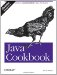# Recipe 5.7 Rounding Floating-Point Numbers

#### Problem

You need to round floating-point numbers to integers or to a particular precision.

#### Solution

If you simply cast a floating value to an integer value, Java truncates the value. A value like 3.999999 cast to an int or long becomes 3, not 4. To round floating-point numbers properly, use Math.round( ) . It has two forms: if you give it a double, you get a long result; if you give it a float, you get an int.

What if you don't like the rounding rules used by round? If for some bizarre reason you wanted to round numbers greater than 0.54 instead of the normal 0.5, you could write your own version of round( ):

`/*  * Round floating values to integers.  * @Return the closest int to the argument.  * @param d A non-negative values to be rounded.  */ static int round(double d) {     if (d < 0) {         throw new IllegalArgumentException("Value must be non-negative");     }     int di = (int)Math.floor(d);    // integral value below (or ==) d     if ((d - di) > THRESHOLD) {         return di + 1;     } else {         return di;     } }`

If you need to display a number with less precision than it normally gets, you probably want to use a DecimalFormat object or a Formatter object.Java Cookbook, Second Edition
ISBN: 0596007019
EAN: 2147483647
Year: 2003
Pages: 409
Authors: Ian F Darwin

Similar book on Amazon